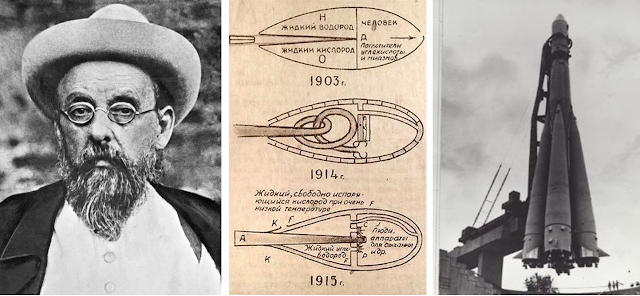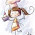### The Tyranny of the Rocket Equation

Today's post is about one of the most fundamental problems in rocket science, both the background of the man who first outlined it and a mathematical example to walk you through it. Launching a rocket presents us with a catch-22 known as "the tyranny of the rocket equation": the rocket and payload have mass, so we need fuel to launch it. But the fuel itself has mass, so we need more fuel to launch the fuel, and more fuel to launch that fuel... I think you see the problem. So how do we get around this?The LEGO model of the Saturn V clearly displays its stages

The solution is to build rocket stages, each containing its own rocket engines and propellant. A stage is jettisoned when it runs out of fuel, saving the weight of the depleted section of the rocket, while the next stage fires its engine and continues accelerating the now-smaller rocket. We know this now and have applied it in practice for decades, but the solution was first clearly laid out by an interesting dude named Konstantin Tsiolkovsky way back in 1896Amazing to think this crusty old bat made space travel possible! Compare his early rocket sketches next to the Soviet Vostok rocket that put the first man in space 60 years later

By all accounts, Tsiolkovsky was a bizarre recluse; he spent most of his early life in a log cabin on the outskirts of Kaluga, about 100 miles southwest of Moscow, reading books about math and physics and becoming fascinated with the idea of space travel. He wrote hundreds of papers detailing technologies decades ahead of its time, such as steering boosters, space stations, airlocks for extravehicular activity, and closed-cycle biological ecosystems to support interplanetary space colonies. One particularly cool fact I found about him - he built Russia's first aerodynamics laboratory in his own apartment, even constructing his own wind tunnel!Google's search bar design in honor of Tsiolkovsky's 155th birthday, on September 17, 2012

Many criticized his works as impractical, and Tsiolkovsky himself never expected his designs to be implemented. His work got more recognition only later in life, and when he died he bequeathed his entire life's work to the Soviet state, directly influencing the top rocket scientists of the Space Race, Wernher von Braun and Sergei Korolev. In retrospect, Tsiolkovsky could be considered the world's first advocate of spaceflight, as he came to believe that "colonizing space would lead to the perfection of the human species, with immortality and a carefree existence." I may not go that far, but no doubt in my mind that our planet is made better by our endeavors beyond it

#### The Tsiolkovsky Rocket Equation

This example is taken directly from Wikipedia and demonstrates the fuel savings of a two-stage orbital rocket versus single-stage. I've adapted it to provide more explanation and make it easier to understand - if you're a nerd like me and have tried learning physics from Wikipedia, you'll know just how intimidating it can be. But I'm just a lowly investment banker, so if I can understand the math behind this, then anyone can!

The Tsiolkovsky rocket equation is given below: it relates a rocket's maximum change in velocity with the effective exhaust velocity and the initial and final masses of the rocket

$\Delta v = v_e \cdot ln(\frac{m_0}{m_f})$

$\Delta v =$ maximum change in velocity
$v_e =$ effective exhaust velocity
$m_0 =$ initial mass including propellant
$m_f =$ final mass without propellant (aka dry mass)

The equation can be rearranged into the following form by dividing both sides by $v_e$ and exponentiating everything by $e$ to give:

$\frac{m_0}{m_f} = e^{\Delta v / v_e}$

Since the difference between $m_0$ and $m_f$ is the propellant that was combusted and expelled from the rocket, this form of the equation is handy because it tells us what proportion of the rocket needs to be propellant. We'll apply this to solve the two cases below

#### Case 1 - Single-Stage Orbital Rocket

Assume a single stage rocket produces effective exhaust velocity of 4,500 m/s and needs to reach a delta-v of 9,700 m/s in order to reach Low Earth Orbit. What percentage of the initial mass needs to be propellant?

$\frac{m_0}{m_f} = e^{9700/4500} = 8.633$

But we're looking for $\frac{m_f}{m_0}$ since the final mass over the initial mass tells us what proportion of the rocket is dry mass

$\frac{m_f}{m_0} = \frac{1}{8.633} = 11.6\%$

$1 - 11.6\% = 88.4\%$

88.4% of the initial mass of the rocket is propellant

The above steps demonstrate algebraically that $\frac{m_0 - m_f}{m_0} = 1-e^{-\Delta v / v_e}$. We'll go ahead and use this shorter formula for the next problem to keep it more concise

#### Case 2 - Two-Stage Orbital Rocket

Now assume the rocket has two stages. The first stage will get us to a delta-v of 5,000 m/s, and the second stage will get us the remaining 4,700 m/s. Also, assume that the dry mass of the first stage alone is 8% of the total mass. Exhaust velocity is still 4,500 m/s

$\frac{m_0 - m_f}{m_0} = 1-e^{-5000/4500} = 67.1\%$

The first stage is accelerating the rocket to 5,000 m/s, so 67.1% of the total rocket's mass is the propellant of the first stage

$100\% - 67.1\% - 8\% = 24.9\%$

67.1% of the rocket's total mass just got expelled as burned propellant. Then, when the first stage jettisoned, its dry mass (given as 8%) is also dropped, so we know that the second stage is equal to 24.9% of the total mass of the rocket

$\frac{m_0 - m_f}{m_0} = 1-e^{-4700/4500} = 64.8\%$

$64.8\% \cdot 24.9\% = 16.1\%$

64.8% of the remaining weight (second stage only) must be propellant, which is 16.1% the weight of the original rocket before the first stage was jettisoned.

$67.1\% + 16.1\% = 83.2\%$

64.8% of the weight of the total rocket is the propellant of the first stage, and 16.1% is the propellant of the second stage.

Now we have our answer: 83.2% of the mass of the original rocket must be propellant, a 5.2% improvement over the 88.4% needed for the single stage rocket

#### Deriving the Tsiolkovsky Rocket Equation

Back when I was in school, it generally annoyed me when we repeatedly used formulas but were given no context on how those formulas were derived. I get that sometimes it's necessary because the derivation is too advanced and beyond the scope of the course (case in point: the Chain Rule from AP Calculus). But I read the derivation of the Tsiolkovsky Rocket Equation from the same Wikipedia page and was pleasantly surprised to find I ultimately understood it as well (albeit with a lot of effort). Although it's more complicated and takes a bit of calculus, if you're curious, check out my explanation below!

And if you're truly insane, Wikipedia has a derivation of the rocket equation that factors in Einstein's theory of Special Relativity. I didn't even bother with this one - good luck!!

1.1.